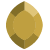# Diurnal correction formulaPosts: 34What's the formula used for Geosoft Oasis Montaj to calculate the diurnal correction?

• Hi @geosoftdehispa to calculate the diurnal correction, you subtract the established magnetic base station value from your daily readings.

With channel math you would use the following expression or formula:

C0 = C1 - 50000

C0 = diurnal correction
5000 = this is a constant and can be replaced with your own established base station value

For subscribed users, check out the following learning path for more info:

Community Manager•Posts: 34That formula doesn't work if the base station is different.
• Hi @geosoftdehispa I don't know what you mean by that. Are you using the Base Station correction tool? You would import the base station data into a new database. Calculate the correction based on the above formula and then export the Date, Time, Correction channels into a Geosoft Table file and run the base station correction tool on your survey data. If you are dealing with a non-typical base station file, you can email [email protected] to get help with a more specific problem.
Community Manager•Posts: 34If I work with that formula, the results will be a relative to the value of the base station won't be the absolute value to the point.
• Hi @geosoftdehispa what do you mean "absolute value to the point"? Does this mean you know the absolute value of the magnetic field at your base station? If this is the case that's fine, you would still do the same calculation to determine the changes in the magnetic field at your base station over the given time period of your survey, but the constant value you remove is the absolute value.
Community Manager•Posts: 34I mean, that the values correcting by one base station dont can be compared with other values of other base station, even doing the IGRF correction.
Exist a way to calculate the absolute value of the magnetic field at my base station.
This discussion has been closed.